# Intermediate Algebra Tutorial 12

Intermediate Algebra
Tutorial 12: Graphing Equations

WTAMU > Virtual Math Lab > Intermediate AlgebraLearning Objectives

After completing this tutorial, you should be able to:
1. Plot points on a rectangular coordinate system.
2. Identify what quadrant or axis a point lies on.
3. Know if an equation is a linear equation.
4. Tell if an ordered pair is a solution of an equation in two variables or not.
5. Graph an equation by plotting points.Introduction

This section covers the basic ideas of graphing:  rectangular coordinate system, solutions to equations in two variables,  and sketching a graph.  Graphs are important in giving a visual representation of the correlation between two variables.  Even though in this section we are going to look at it generically, using a general x and y variable, you can use two-dimensional graphs for any application where you have two variables.  For example, you may have a cost function that is dependent on the quantity of items made.  If you needed to show your boss visually the correlation of the quantity with the cost, you could do that on a two-dimensional graph.  I believe that it is important for you learn how to do something in general, then when you need to apply it to something specific you have the knowledge to do so.  Going from general to specific is a lot easier than specific to general.  And that is what we are doing here looking at graphing in general so later you can apply it to something specific, if needed.Tutorial

Rectangular Coordinate System

The following is the rectangular coordinate system: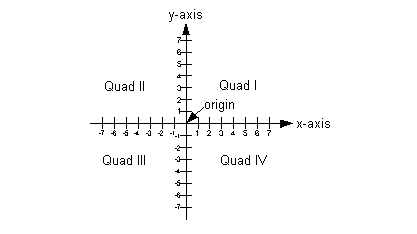It is made up of two number lines:
1. The horizontal number line is the x- axis.
2. The vertical number line is the y- axis.
The origin is where the two intersect.  This is where both number lines are 0.

It is split into four quadrants which are marked on this graph with Roman numerals.

Each point on the graph is associated with an ordered pair.  When dealing with an x, y graph, x is always first and y is always second in the ordered pair (x, y).  It is a solution to an equation in two variables.  Even though there are two values in the ordered pair, be careful that it associates to ONLY ONE point on the graph,  the point lines up with both the x value of the ordered pair (x-axis) and the y value of the ordered pair (y-axis).Example 1:    Plot the ordered pairs and name the quadrant or axis in which the point lies.     A(2, 3), B(-1, 2), C(-3, -4), D(2, 0), and E(0, 5).

Remember that each ordered pair associates with only one point on the graph.  Just line up the x value and then the y value to get your location.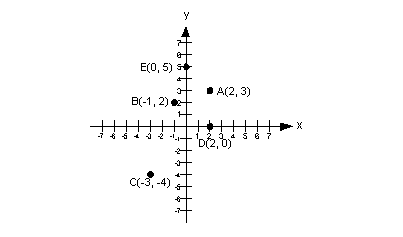A(2, 3) lies in quadrant I.

B(-1, 2) lies in quadrant II.

C(-3, -4) lies in quadrant III.

D(2, 0) lies on the x-axis.

E(0, 5) lies on the y-axis.

Solutions of Equations
in Two Variables

The solutions to equations in two variables consist of two values that when substituted into their corresponding variables in the equation, make a true statement.

In other words, if your equation has two variables x and y, and you plug in a value for x and its corresponding value for y and the mathematical statement comes out to be true, then the x and y value that you plugged in would together be a solution to the equation.

Equations in two variables can have more than one solution.

We usually write the solutions to equations in two variables in ordered pairs.Example 2:  Determine whether each ordered pair is a solution of the given equation.     y = 5x - 7;  (2, 3), (1, 5), (-1, -12)

Which number is the x value and which one is the y value?  If you said x = 2 and y = 3, you are correct!

Let’s plug (2, 3) into the equation and see what we get: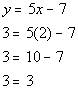*Plug in 2 for x and 3 for y

This is a TRUE statement, so (2, 3) is a solution to the equation y = 5x - 7.

Now let’s take a look at (1, 5).

Which number is the x value and which one is the y value?    If you said x = 1 and y = 5, you are right!

Let’s plug (1, 5) into the equation and see what we get: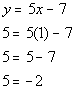*Plug in 1 for x and 5 for y

Whoops, it looks like we have ourselves a FALSE statement. This means that (1, 5) is NOT a solution to the equation 5x - 7.

Now let’s look at (-1, -12).

Which number is the x value and which one is the y value?    If you said x = -1 and y = -12, you are right!

Let’s plug (-1, -12) into the equation and see what we get: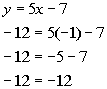*Plug in -1 for x and -12 for y

We have another TRUE statement.  This means (-1, -12) is another solution to the equation y = 5x - 7.

Note that you were only given three ordered pairs to check, however, there are an infinite number of solutions to this equation.  It would very cumbersome to find them all.Example 3:  Determine whether each ordered pair is a solution of the given equation.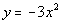;  (0, -3), (1, -3), (-1, -3)

Which number is the x value and which one is the y value?  If you said x = 0 and y = -3, you are correct!

Let’s plug (0, -3) into the equation and see what we get: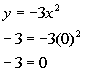*Plug in 0 for x and -3 for y

This is a FALSE statement, so (0, -3) is NOT a solution to the equationNow, let’s take a look at (1, -3).

Which number is the x value and which one is the y value?    If you said x = 1 and y = -3, you are right!

Let’s plug (1, -3) into the equation and see what we get: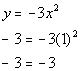*Plug in 1 for x and -3 for y

This is a TRUE statement. This means that (1, -3) is a solution to the equation.

Now, let’s look at (-1, -3).

Which number is the x value and which one is the y value?    If you said x = -1 and y = -3, you are right!

Let’s plug (-1, -3) into the equation and see what we get: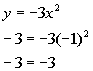*Plug in -1 for x and -3 for y

This is a TRUE statement. This means that (1, -3) is a solution to the equation.

Linear Equation in
Two Variables

Standard Form:
Ax + By = C

A linear equation in two variables is an equation that can be written in the form Ax + By = C,  where A and B are not both 0.

This form is called the standard form of a linear equation.Example 4:  Write the following linear equation in standard form.
y = 7x - 5

This means we want to write it in the form Ax + By = C.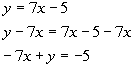*Inverse of add 7x is sub. 7x
*In standard form

Graphing a Linear Equation
by Plotting Points

If the equation is linear:

Step 1:  Find three ordered pair solutions.

You do this by plugging in ANY three values for x and find their corresponding y values.

Yes, it can be ANY three values you want, 1, -3, or even 10,000.  Remember there are an infinite number of solutions.  As long as you find the corresponding y value that goes with each x, you have a solution.

Step 2:  Plot the points found in step 1.

Remember that each ordered pair corresponds to only one point on the graph.

The point lines up with both the x value of the ordered pair (x-axis) and the y value of the ordered pair (y-axis).

Step 3:  Draw the graph.

A linear equation will graph as a straight line.

If you know it is a linear equation and your points don’t line up, then you either need to check your math in step 1 and/or that you plotted all the points found correctly.Example 5:   Determine whether the equation is linear or not.  Then graph the equation.    y = 5x - 3

If we subtract 5x from both sides, then we can write the given equation as -5x + y = -3.

Since we can write it in the standard form, Ax + By = C, then we have a linear equation.

This means that we will have a line when we go to graph this.

Step 1:  Find three ordered pair solutions.

I’m going to use a chart to organize my information.  A  chart keeps track of the x values that you are using and the corresponding y value found when you used a particular x value.

If you do this step the same each time, then it will make it easier for you to remember how to do it.

I usually pick out three points when I know I’m dealing with a line.  The three x values I’m going to use are -1, 0, and 1.  (Note that you can pick ANY three x values that you want.  You do not have to use the values that I picked.)  You want to keep it as simple as possible.  The following is the chart I ended up with after plugging in the values I mentioned for x.

x y = 5x - 3 (x, y) -1 y = 5(-1) - 3 = -8 (-1, -8) 0 y = 5(0) - 3 = -3 (0, -3) 1 y = 5(1) - 3 = 2 (1, 2)

Step 2:  Plot the points found in step 1.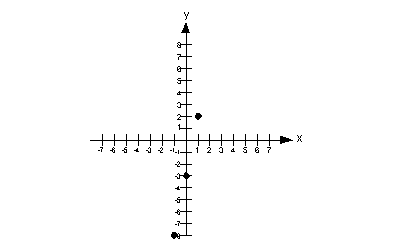Step 3:  Draw the graph.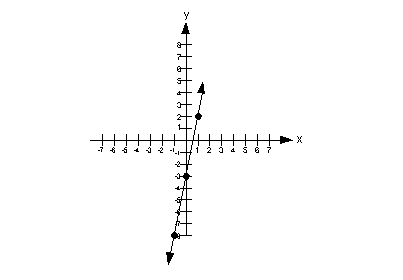Graphing a Non-Linear Equation
by Plotting Points

If the equation is non-linear:

Step 1:  Find six or seven ordered pair solutions.

Non-linear equations can vary on what the graph looks like.  So it is good to have a lot of points so you can get the right shape of the graph.

Step 2:  Plot the points found in step 1.

Step 3:  Draw the graph.

If the points line up draw a straight line through them.  If the points are in a curve, draw a curve through them.Example 6:   Determine whether the equation is linear or not.  Then graph the equation.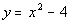If we subtract the x squared from both sides, we would end up with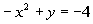.   Is this a linear equation?  Note how we have an x squared as opposed to x to the one power.

It looks like we cannot write it in the form Ax + By = C because the x has to be to the one power, not squared.  So this is not a linear equation.

However, we can still graph it.

Step 1:  Find six or seven ordered pair solutions.

The seven x values that I'm going to use are -3, -2, -1, 0, 1, 2, and 3.  (Note that you can pick ANY x values that you want.  You do not have to use the values that I picked.) You want to keep it as simple as possible.  The following is the chart I ended up with after plugging in the values I mentioned for x

Note that the carrot top (^) represents an exponent. For example, x squared can be written as x^2.  The second column is showing you the 'scratch work' of how we got the corresponding value of y.

x(x, y) -3 y = (-3)^2 - 4 = 5 (-3, 5) -2 y = (-2)^2 - 4 = 0 (-2, 0) -1 y = (-1)^2 - 4 = -3 (-1, -3) 0 y = (0)^2 - 4 = -4 (0, -4) 1 y = (1)^2 - 4 = -3 (1, -3) 2 y = (2)^2 - 4 = 0  (2, 0) 3 y = (3)^2 - 4 = 5 (3, 5)

Step 2:  Plot the points found in step 1.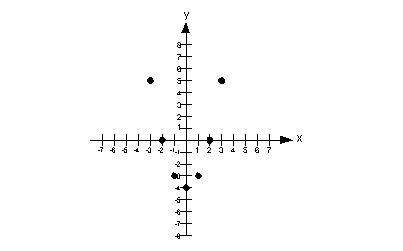Step 3:  Draw the graph.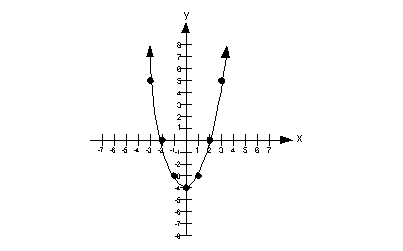Example 7:   Determine whether the equation is linear or not.  Then graph the equation.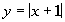Do you think this equation is linear or not?  It is a tricky problem because both the x and y variables are to the one power.  However, x is inside the absolute value sign and we can’t just take it out of there.

In other words, we can’t write it in the form Ax + By = C.  This means that this equation is not a linear equation.

If you are unsure that an equation is linear or not, you can ALWAYS plug in x values and find the corresponding y values to come up with ordered pairs to plot.

Step 1:  Find six or seven ordered pair solutions.

The seven x values that I'm going to use are -3, -2, -1, 0, 1, 2, and 3.  (Note that you can pick ANY x values that you want.  You do not have to use the values that I picked.) You want to keep it as simple as possible.  The following is the chart I ended up with after plugging in the values I mentioned for x.

x(x, y) -3 y = |-3 + 1| = 2 (-3, 2) -2 y = |-2 + 1| = 1 (-2, 1) -1 y = |-1 + 1| = 0 (-1, 0) 0 y = |0 + 1| = 1 (0, 1) 1 y = |1 + 1| = 2 (1, 2) 2 y = |2 + 1| = 3 (2, 3) 3 y = |3 + 1| = 4 (3, 4)

Step 2:  Plot the points found in step 1.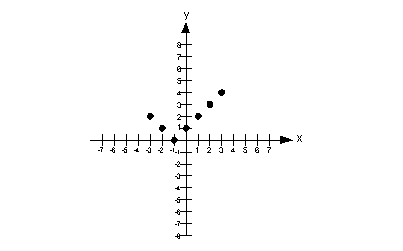Step 3:  Draw the graph.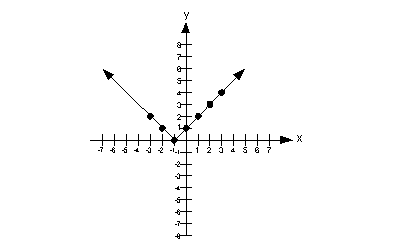Practice Problems

These are practice problems to help bring you to the next level.  It will allow you to check and see if you have an understanding of these types of problems. Math works just like anything else, if you want to get good at it, then you need to practice it.  Even the best athletes and musicians had help along the way and lots of practice, practice, practice, to get good at their sport or instrument.  In fact there is no such thing as too much practice.

To get the most out of these, you should work the problem out on your own and then check your answer by clicking on the link for the answer/discussion for that  problem.  At the link you will find the answer as well as any steps that went into finding that answer.Practice Problem 1a: Plot the ordered pairs and name the quadrant or axis in which the point lies.

1a.  A(3, 1), B(-2, -1/2), C(2, -2), and D(0,1)Practice Problem 2a: Determine if each ordered pair is a solution of the given equation.

2a.    y = 4x - 10     (0, -10), (1, -14), (-1, -14)Practice Problems 3a - 3c: Determine whether each equation is linear or not.  Then graph the equation.

3a.    y = 2x - 1
3b.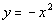3c.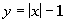Need Extra Help on these Topics?

The following are webpages that can assist you in the topics that were covered on this page:

http://www.purplemath.com/modules/plane.htm
This webpage helps you with plotting points.

http://www.math.com/school/subject2/lessons/S2U4L1DP.html
This website helps you with plotting points.

http://www.purplemath.com/modules/graphlin.htm
This webpage helps you with graphing linear equations.

Go to Get Help Outside the Classroom found in Tutorial 1: How to Succeed in a Math Class for some more suggestions.Last revised on July 3, 2011 by Kim Seward.